$$\newcommand{\id}{\mathrm{id}}$$ $$\newcommand{\Span}{\mathrm{span}}$$ $$\newcommand{\kernel}{\mathrm{null}\,}$$ $$\newcommand{\range}{\mathrm{range}\,}$$ $$\newcommand{\RealPart}{\mathrm{Re}}$$ $$\newcommand{\ImaginaryPart}{\mathrm{Im}}$$ $$\newcommand{\Argument}{\mathrm{Arg}}$$ $$\newcommand{\norm}{\| #1 \|}$$ $$\newcommand{\inner}{\langle #1, #2 \rangle}$$ $$\newcommand{\Span}{\mathrm{span}}$$

2.7: Solving Inequalities with Two Variables

$$\newcommand{\vecs}{\overset { \rightharpoonup} {\mathbf{#1}} }$$

$$\newcommand{\vecd}{\overset{-\!-\!\rightharpoonup}{\vphantom{a}\smash {#1}}}$$

Skills to Develop

• Identify and check solutions to inequalities with two variables.
• Graph solution sets of linear inequalities with two variables.

Solutions to Inequalities with Two Variables

We know that a linear equation with two variables has infinitely many ordered pair solutions that form a line when graphed. A linear inequality with two variables65, on the other hand, has a solution set consisting of a region that defines half of the plane.

Linear Equation Linear Inequality
$$y = \frac { 3 } { 2 } x + 3$$ $$y \leq \frac { 3 } { 2 } x + 3$$Figure 2.7.1Figure 2.7.2

Table 2.7.1

For the inequality, the line defines the boundary of the region that is shaded. This indicates that any ordered pair in the shaded region, including the boundary line, will satisfy the inequality. To see that this is the case, choose a few test points66 and substitute them into the inequality.

 Test Point $$y \leq \frac { 3 } { 2 } x + 3$$ $$( 0,0 )$$ $$\begin{array} { l } { 0 \leq \frac { 3 } { 2 } ( 0 ) + 3 } \\ { 0 \leq 3 } \:\:\:\color{Cerulean}{✓} \end{array}$$ $$( 2,1 )$$ $$\begin{array} { l } { 1 \leq \frac { 3 } { 2 } ( 2 ) + 3 } \\ { 1 \leq 3 + 3 } \\ { 1 \leq 6 }\:\:\:\color{Cerulean}{✓} \end{array}$$ $$( - 2 , - 1 )$$ $$\begin{array} { l } { - 1 \leq \frac { 3 } { 2 } ( - 2 ) + 3 } \\ { - 1 \leq - 3 + 3 } \\ { - 1 \leq 0 } \:\:\:\color{Cerulean}{✓} \end{array}$$

Table 2.7.2

Also, we can see that ordered pairs outside the shaded region do not solve the linear inequality.

 Test Point $$y \leq \frac { 3 } { 2 } x + 3$$ $$(-2,3)$$ $$\begin{array} { l } { 3 \leq \frac { 3 } { 2 } ( - 2 ) + 3 } \\ { 3 \leq - 3 + 3 } \\ { 3 \leq 0 \quad x }\color{red}{✗} \end{array}\ Table 2.7.3 The graph of the solution set to a linear inequality is always a region. However, the boundary may not always be included in that set. In the previous example, the line was part of the solution set because of the “or equal to” part of the inclusive inequality \(≤$$. If given a strict inequality $$<$$, we would then use a dashed line to indicate that those points are not included in the solution set.

Non-inclusive Boundary Inclusive Boundary
$$y < \frac { 3 } { 2 } x + 3$$ $$y \leq \frac { 3 } { 2 } x + 3$$Figure 2.7.3Figure 2.7.4

Table 2.7.4

Consider the point $$(0, 3)$$ on the boundary; this ordered pair satisfies the linear equation. It is the “or equal to” part of the inclusive inequality that makes the ordered pair part of the solution set.

 $$y < \frac { 3 } { 2 } x + 3$$ $$y \leq \frac { 3 } { 2 } x + 3$$ $$\begin{array} { l } { 3 < \frac { 3 } { 2 } ( 0 ) + 3 } \\ { 3 < 0 + 3 } \\ { 3 < 3 x } \color{red}{✗}\end{array}$$ $$\begin{array} { l } { 3 \leq \frac { 3 } { 2 } ( 0 ) + 3 } \\ { 3 \leq 0 + 3 } \\ { 3 \leq 3 }\color{Cerulean}{✓} \end{array}$$

Table 2.7.5

So far we have seen examples of inequalities that were “less than.” Now consider the following graphs with the same boundary:

Greater Than (Above) Less Than (Below)
$$y \geq \frac { 3 } { 2 } x + 3$$ $$y \leq \frac { 3 } { 2 } x + 3$$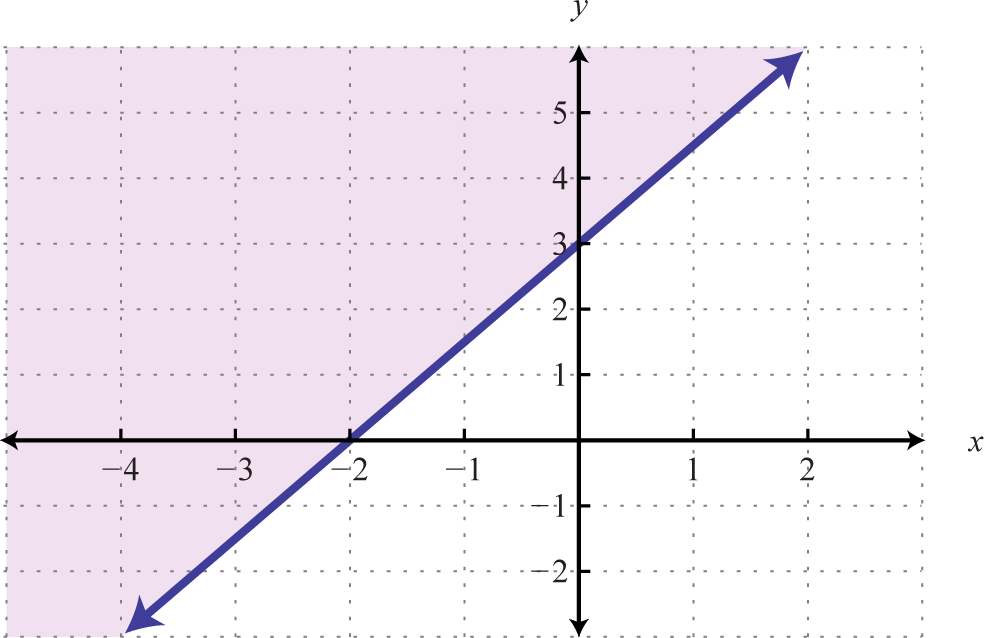Figure 2.7.5Figure 2.7.6

Table 2.7.6

Given the graphs above, what might we expect if we use the origin (0, 0) as a test point?

 $$y \geq \frac { 3 } { 2 } x + 3$$ $$y \leq \frac { 3 } { 2 } x + 3$$ $$\begin{array} { l } { 0 \geq \frac { 3 } { 2 } ( 0 ) + 3 } \\ { 0 \geq 0 + 3 } \\ { 0 \geq 3 } \color{red}{✗} \end{array}$$ $$\begin{array} { l } { 0 \leq \frac { 3 } { 2 } ( 0 ) + 3 } \\ { 0 \leq 0 + 3 } \\ { 0 \leq 3 } \color{Cerulean}{✓} \end{array}$$

Table 2.7.7

Example $$\PageIndex{1}$$:

Determine whether or not $$(2, \frac{1}{2})$$ is a solution to $$5x − 2y < 10$$.

Solution:

Substitute the $$x$$- and $$y$$-values into the equation and see if a true statement is obtained.

$$\begin{array} { r } { 5 x - 2 y < 10 } \\ { 5 ( \color{Cerulean}{2}\color{Black}{ )} - 2 \left( \color{Cerulean}{\frac { 1 } { 2 }} \right) < 10 } \\ { 10 - 1 < 10 } \\ { 9 < 10 } \color{Cerulean}{✓} \end{array}$$

$$(2, \frac{1}{2})$$ is a solution.

These ideas and techniques extend to nonlinear inequalities with two variables. For example, all of the solutions to $$y > x^{2}$$ are shaded in the graph below.Figure 2.7.7

The boundary of the region is a parabola, shown as a dashed curve on the graph, and is not part of the solution set. However, from the graph we expect the ordered pair $$(−1,4)$$ to be a solution. Furthermore, we expect that ordered pairs that are not in the shaded region, such as $$(−3, 2)$$, will not satisfy the inequality.

 Check $$(-1, 4)$$ Check $$(-3, 2)$$ $$\begin{array} { l } { y > x ^ { 2 } } \\ { 4 > ( - 1 ) ^ { 2 } } \\ { 4 > 1 } \color{Cerulean}{✓}\end{array}$$ $$\begin{array} { l } { y > x ^ { 2 } } \\ { 2 > ( - 3 ) ^ { 2 } } \\ { 2 > 9 } \color{red}{✗}\end{array}$$

Table 2.7.8

Following are graphs of solutions sets of inequalities with inclusive parabolic boundaries.

 $$y \leq ( x - 1 ) ^ { 2 } - 2$$ $$y \geq ( x - 1 ) ^ { 2 } - 2$$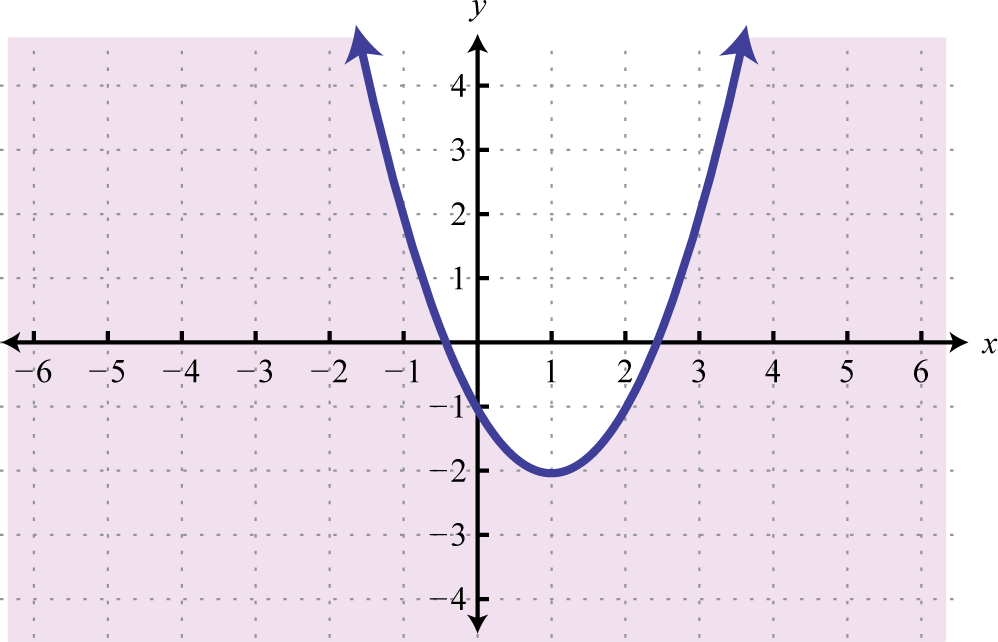Figure 2.7.8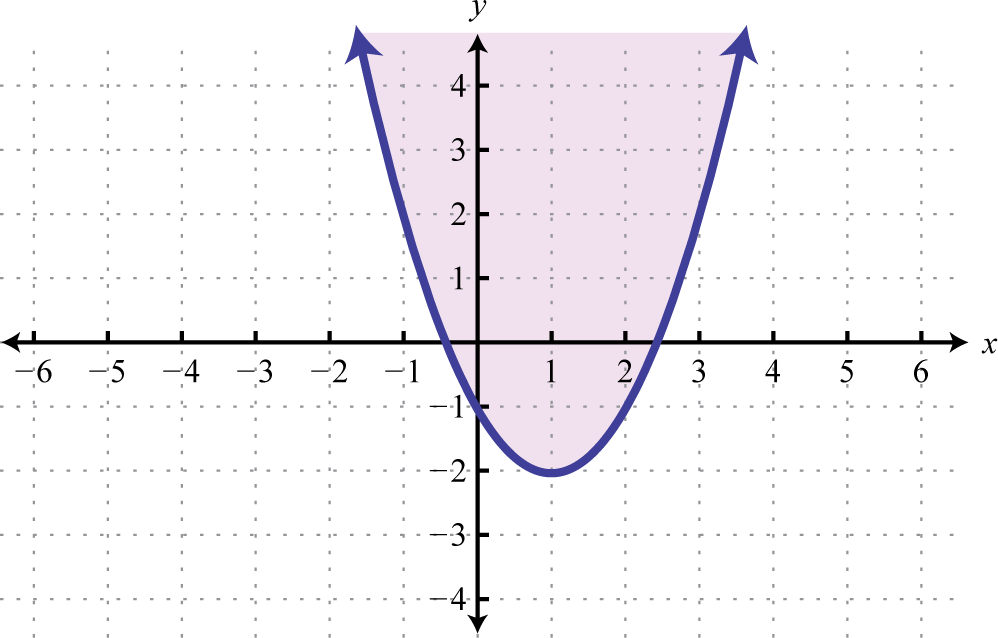Figure 2.7.9

Table 2.7.9

You are encouraged to test points in and out of each solution set that is graphed above.

Exercise $$\PageIndex{1}$$

Is $$(-3, -2)$$ a solution to $$2x-3y<0$$?

No

Graphing Solutions to Inequalities with Two Variables

Solutions to linear inequalities are a shaded half-plane, bounded by a solid line or a dashed line. This boundary is either included in the solution or not, depending on the given inequality. If we are given a strict inequality, we use a dashed line to indicate that the boundary is not included. If we are given an inclusive inequality, we use a solid line to indicate that it is included. The steps for graphing the solution set for an inequality with two variables are shown in the following example.

Example $$\PageIndex{2}$$:

Graph the solution set $$y>−3x+1$$.

Solution:

• Step 1: Graph the boundary. Because of the strict inequality, we will graph the boundary $$y=−3x+1$$ using a dashed line. We can see that the slope is $$m=−3=−\frac{3}{1}=\frac{rise}{run}$$ and the $$y$$-intercept is $$(0, 1)$$.Figure 2.7.10

• Step 2: Test a point that is not on the boundary. A common test point is the origin, $$(0, 0)$$. The test point helps us determine which half of the plane to shade.

 Test Point $$y > - 3 x + 1$$ $$(0,0)$$ $$\begin{array} { l } { 0 > - 3 ( 0 ) + 1 } \\ { 0 > 1 }\color{red}{✗} \end{array}$$
Table 2.7.10
• Step 3: Shade the region containing the solutions. Since the test point $$(0, 0)$$ was not a solution, it does not lie in the region containing all the ordered pair solutions. Therefore, shade the half of the plane that does not contain this test point. In this case, shade above the boundary line.Figure 2.7.11

Consider the problem of shading above or below the boundary line when the inequality is in slope-intercept form. If $$y > mx + b$$, then shade above the line. If $$y < mx + b$$, then shade below the line. Shade with caution; sometimes the boundary is given in standard form, in which case these rules do not apply.

Example $$\PageIndex{3}$$:

Graph the solution set $$2 x - 5 y \geq - 10$$.

Solution:

Here the boundary is defined by the line $$2x − 5y = −10$$. Since the inequality is inclusive, we graph the boundary using a solid line. In this case, graph the boundary line using intercepts.

 To find the $$x$$-intercept, set $$y = 0$$. To find the $$y$$-intercept, set $$x = 0$$. $$\begin{array} { c } { 2 x - 5 y = - 10 } \\ { 2 x - 5 ( \color{Cerulean}{0}\color{Black}{ )} = - 10 } \\ { 2 x = - 10 } \\ { x = - 5 } \end{array}$$ $$\begin{array} { r } { 2 x - 5 y = - 10 } \\ { 2 ( \color{Cerulean}{0}\color{Black}{ )} - 5 y = - 10 } \\ { - 5 y = - 10 } \\ { y = 2 } \end{array}$$ $$x$$-intercept: $$(−5, 0)$$ $$y$$-intercept: $$(0, 2)$$

Table 2.7.11Figure 2.7.12

Next, test a point; this helps decide which region to shade.

 Test Point $$2 x - 5 y \geq - 10$$ $$(0,0)$$ \begin{aligned} 2 ( 0 ) - 5 ( 0 ) & \geq - 10 \\ 0 & \geq - 10 \color{Cerulean}{✓}\end{aligned}

Table 2.7.12

Since the test point is in the solution set, shade the half of the plane that contains it.Figure 2.7.13

In this example, notice that the solution set consists of all the ordered pairs below the boundary line. This may seem counterintuitive because the original inequality involved “greater than” $$≥$$. This illustrates that it is a best practice to actually test a point. Solve for $$y$$ and you see that the shading is correct.

\begin{aligned} 2 x - 5 y & \geq - 10 \\ 2 x - 5 y \color{Cerulean}{- 2 x} & \geq - 10 \color{Cerulean}{-2x} \\ - 5 y & \geq - 2 x - 10 \\ \frac { - 5 y } { \color{Cerulean}{- 5} } & \color{OliveGreen}{\leq} \color{Black}{\frac { - 2 x - 10 } { \color{Cerulean}{- 5} }} \:\:\:\color{Cerulean}{Reverse\: the\: inequality.}\\ y & \leq \frac { 2 } { 5 } x + 2 \end{aligned}

In slope-intercept form, you can see that the region below the boundary line should be shaded. An alternate approach is to first express the boundary in slope-intercept form, graph it, and then shade the appropriate region.

Example $$\PageIndex{4}$$:

Graph the solution set $$y <2$$.

Solution:

First, graph the boundary line $$y = 2$$ with a dashed line because of the strict inequality. Next, test a point.

 Test Point $$y<2$$ $$(0,0)$$ $$0 < 2\color{Cerulean}{✓}$$

Table 2.7.13

In this case, shade the region that contains the test point.Figure 2.7.14

Exercise $$\PageIndex{2}$$

Graph the solution set $$2 x - 3 y < 0$$.Figure 2.7.15

The steps are the same for nonlinear inequalities with two variables. Graph the boundary first and then test a point to determine which region contains the solutions.

Example $$\PageIndex{5}$$:

Graph the solution set $$y < ( x + 2 ) ^ { 2 } - 1$$.

Solution:

The boundary is a basic parabola shifted $$2$$ units to the left and $$1$$ unit down. Begin by drawing a dashed parabolic boundary because of the strict inequality.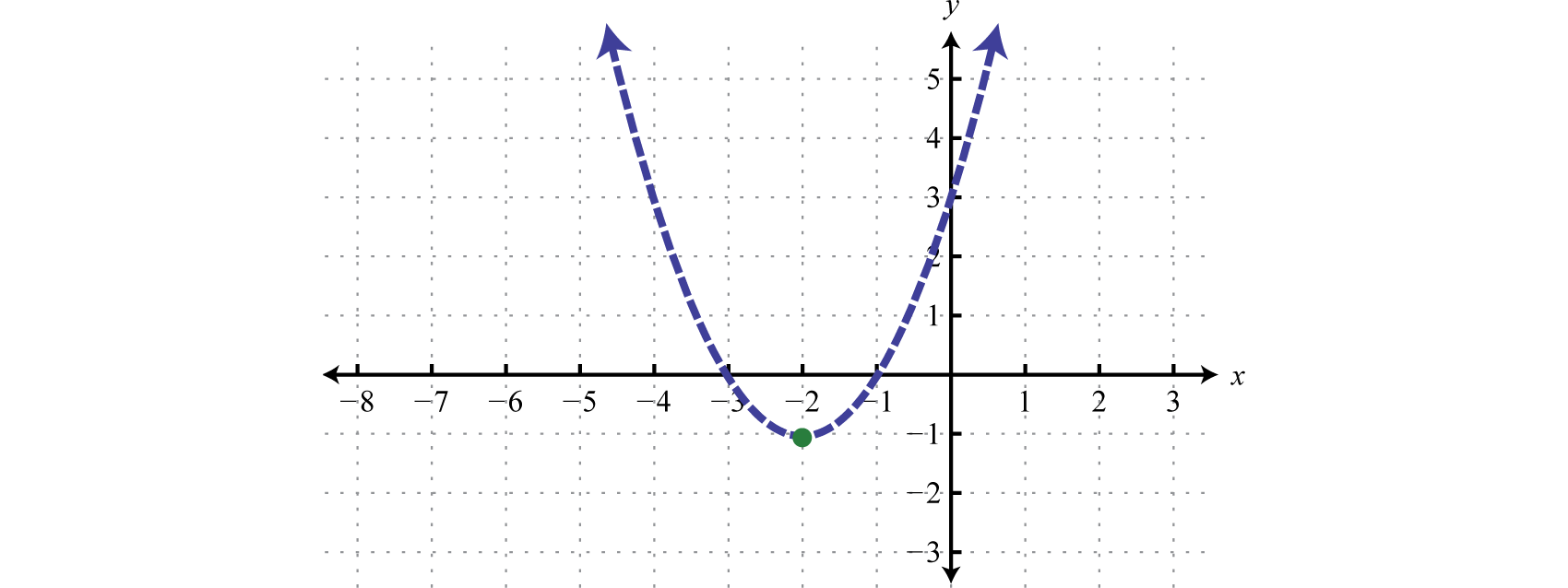Figure 2.7.16

Next, test a point.

 Test Point $$y < ( x + 2 ) ^ { 2 } - 1$$ $$(0,0)$$ $$\begin{array} { l } { 0 < ( 0 + 2 ) ^ { 2 } - 1 } \\ { 0 < 4 - 1 } \\ { 0 < 3 } \color{Cerulean}{✓}\end{array}$$

Table 2.7.14

In this case, shade the region that contains the test point $$(0, 0)$$.Figure 2.7.17

Example $$\PageIndex{6}$$:

Graph the solution set $$y \geq x ^ { 2 } + 3$$.

Solution:

The boundary is a basic parabola shifted $$3$$ units up. It is graphed using a solid curve because of the inclusive inequality.Figure 2.7.18

Next, test a point.

 Test Point $$y \geq x ^ { 2 } + 3$$ $$(0,0)$$ $$\begin{array} { l } { 0 \geq 0 ^ { 2 } + 3 } \\ { 0 \geq 3 } \color{red}{✗} \end{array}$$

Table 2.7.15

In this case, shade the region that does not contain the test point $$(0,0)$$.Figure 2.7.19

Exercise $$\PageIndex{3}$$

Graph the solution set $$y < | x - 1 | - 3$$.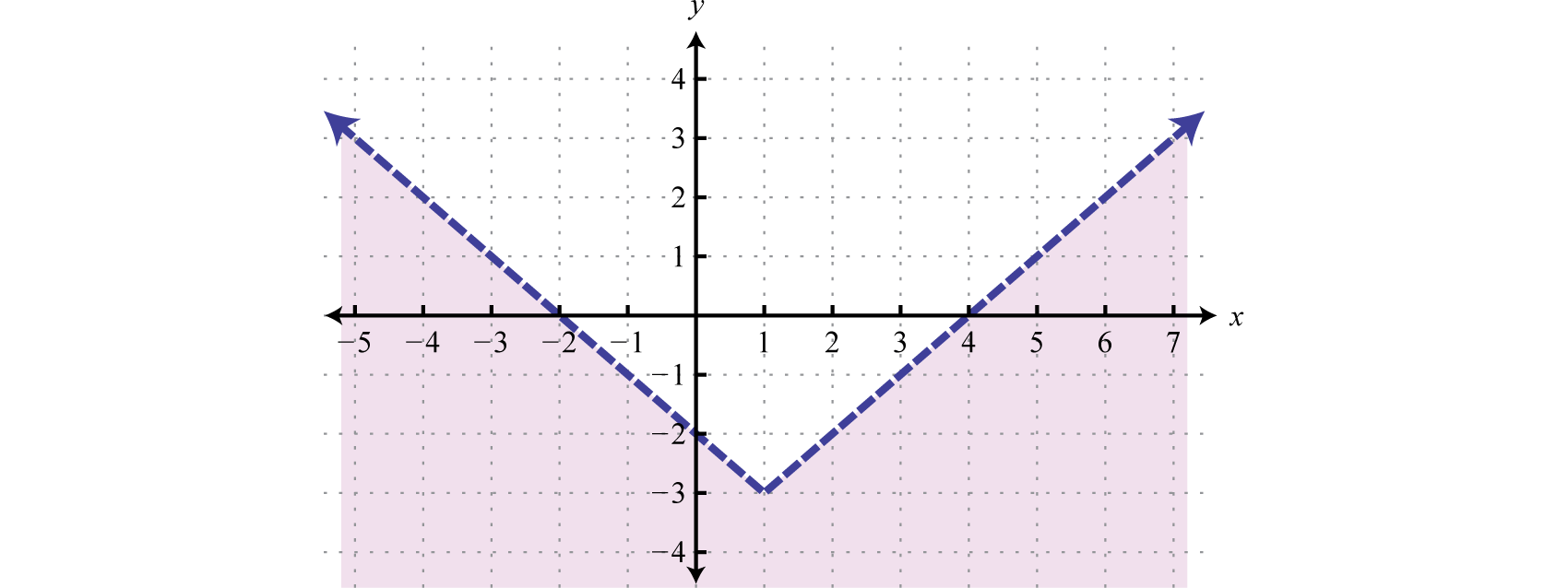Figure 2.7.20

Key Takeaways

• Linear inequalities with two variables have infinitely many ordered pair solutions, which can be graphed by shading in the appropriate half of a rectangular coordinate plane.
• To graph the solution set of an inequality with two variables, first graph the boundary with a dashed or solid line depending on the inequality. If given a strict inequality, use a dashed line for the boundary. If given an inclusive inequality, use a solid line. Next, choose a test point not on the boundary. If the test point solves the inequality, then shade the region that contains it; otherwise, shade the opposite side.
• Check your answer by testing points in and out of the shading region to verify that they solve the inequality or not.

Exercise $$\PageIndex{4}$$

Is the ordered pair a solution to the given inequality?

1. $$5 x - y > - 2 ; ( - 3 , - 4 )$$
2. $$4 x - y < - 8 ; ( - 3 , - 10 )$$
3. $$6 x - 15 y \geq - 1 ; \left( \frac { 1 } { 2 } , - \frac { 1 } { 3 } \right)$$
4. $$x - 2 y \geq 2 ; \left( \frac { 2 } { 3 } , - \frac { 5 } { 6 } \right)$$
5. $$\frac { 3 } { 4 } x - \frac { 2 } { 3 } y < \frac { 3 } { 2 } ; ( 1 , - 1 )$$
6. $$\frac { 2 } { 5 } x + \frac { 4 } { 3 } y > \frac { 1 } { 2 } ; ( - 2,1 )$$
7. $$y \leq x ^ { 2 } - 1 ; ( - 1,1 )$$
8. $$y \geq x ^ { 2 } + 3 ; ( - 2,0 )$$
9. $$y \geq ( x - 5 ) ^ { 2 } + 1 ; ( 3,4 )$$
10. $$y \leq 2 ( x + 1 ) ^ { 2 } - 3 ; ( - 1 , - 2 )$$
11. $$y > 3 - | x | ; ( - 4 , - 3 )$$
12. $$y < | x | - 8 ; ( 5 , - 7 )$$
13. $$y > | 2 x - 1 | - 3 ; ( - 1,3 )$$
14. $$y < | 3 x - 2 | + 2 ; ( - 2,10 )$$

1. No

3. Yes

5. Yes

7. No

9. No

11. No

13. Yes

Exercise $$\PageIndex{5}$$

Graph the solution set.

1. $$y < 2 x - 1$$
2. $$y > −4x + 1$$
3. $$y ≥ −\frac{2}{3} x + 3$$
4. $$y ≤ \frac{4}{3} x − 3$$
5. $$2x + 3y ≤ 18$$
6. $$5x + 2y ≤ 8$$
7. $$6x − 5y > 30$$
8. $$8x − 6y < 24$$
9. $$3x − 4y < 0$$
10. $$x − 3y > 0$$
11. $$x + y ≥ 0$$
12. $$x − y ≥ 0$$
13. $$y ≤ −2$$
14. $$y > −3$$
15. $$x < −2$$
16. $$x ≥ −3$$
17. $$\frac { 1 } { 6 } x + \frac { 1 } { 10 } y \leq \frac { 1 } { 2 }$$
18. $$\frac { 3 } { 8 } x + \frac { 1 } { 2 } y \geq \frac { 3 } { 4 }$$
19. $$\frac { 1 } { 12 } x - \frac { 1 } { 6 } y < \frac { 2 } { 3 }$$
20. $$\frac { 1 } { 3 } x - \frac { 1 } { 9 } y > \frac { 4 } { 3 }$$
21. $$5x ≤ −4y − 12$$
22. $$−4x ≤ 12 − 3y$$
23. $$4y + 2 < 3x$$
24. $$8x < 9 − 6y$$
25. $$5 ≥ 3x − 15y$$
26. $$2 x \geq 6 - 9 y$$
27. Write an inequality that describes all points in the upper half-plane above the $$x$$-axis.
28. Write an inequality that describes all points in the lower half-plane below the $$x$$-axis.
29. Write an inequality that describes all points in the half-plane left of the $$y$$-axis.
30. Write an inequality that describes all points in the half-plane right of the $$y$$-axis.
31. Write an inequality that describes all ordered pairs whose $$y$$-coordinate is at least $$k$$ units.
32. Write an inequality that describes all ordered pairs whose $$x$$-coordinate is at most $$k$$ units.

1.Figure 2.7.21

3.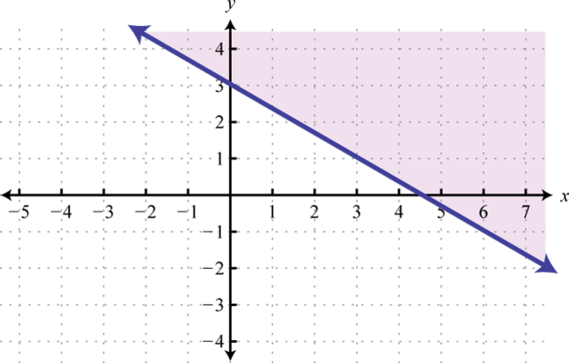Figure 2.7.22

5.Figure 2.7.23

7.Figure 2.7.24

9.Figure 2.7.25

11.Figure 2.7.26

13.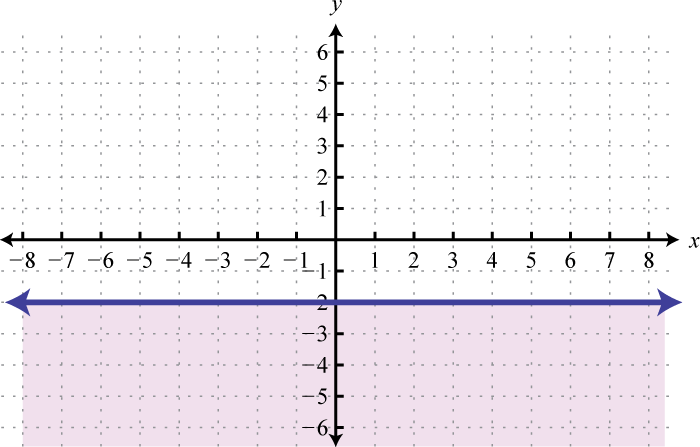Figure 2.7.27

15.Figure 2.7.28

17.Figure 2.7.29

19.Figure 2.7.30

21.Figure 2.7.31

23.Figure 2.7.32

25.Figure 2.7.33

27. $$y > 0$$

29. $$x < 0$$

31. $$y \geq k$$

Exercise $$\PageIndex{6}$$

Graph the solution set.

1. $$y ≤ x^{2} + 3$$
2. $$y > x^{2} − 2$$
3. $$y ≤ −x^{2}$$
4. $$y ≥ −x^{2}$$
5. $$y > (x + 1)^{2}$$
6. $$y > (x − 2)^{2}$$
7. $$y ≤ (x − 1)^{2} + 2$$
8. $$y ≤ (x + 3)^{2} − 1$$
9. $$y < −x^{2} + 1$$
10. $$y > −(x − 2)^{2} + 1$$
11. $$y ≥ |x| − 2$$
12. $$y < |x| + 1$$
13. $$y < |x − 3|$$
14. $$y ≤ |x + 2|$$
15. $$y > − |x + 1|$$
16. $$y ≤ − |x − 2|$$
17. $$y ≥ |x + 3| − 2$$
18. $$y ≥ |x − 2| − 1$$
19. $$y < − |x + 4| + 2$$
20. $$y > − |x − 4| − 1$$
21. $$y > x^{3} − 1$$
22. $$y ≤ x^{3} + 2$$
23. $$y ≤ \sqrt{x}$$
24. $$y > \sqrt{x}− 1$$
25. A rectangular pen is to be constructed with at most $$200$$ feet of fencing. Write a linear inequality in terms of the length $$l$$ and the width $$w$$. Sketch the graph of all possible solutions to this problem.
26. A company sells one product for $$8$$ and another for $$12$$. How many of each product must be sold so that revenues are at least $$2,400$$? Let $$x$$ represent the number of products sold at $$8$$ and let $$y$$ represent the number of products sold at $$12$$. Write a linear inequality in terms of $$x$$ and $$y$$ and sketch the graph of all possible solutions.

1.Figure 2.7.34

3.Figure 2.7.35

5.Figure 2.7.36

7.Figure 2.7.37

9.Figure 2.7.38

11.Figure 2.7.39

13.Figure 2.7.40

15.Figure 2.7.41

17.Figure 2.7.42

19.Figure 2.7.43

21.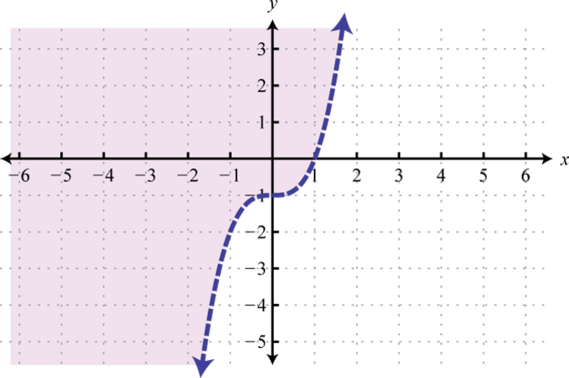Figure 2.7.44

23.Figure 2.7.45

25. $$l + w \leq 100$$;Figure 2.7.46

Footnotes

65An inequality relating linear expressions with two variables. The solution set is a region defining half of the plane.

66A point not on the boundary of the linear inequality used as a means to determine in which half-plane the solutions lie.